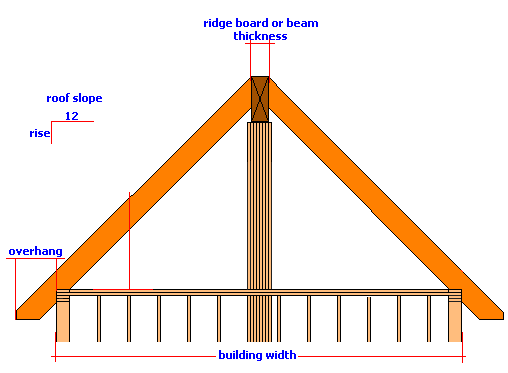# Rafter Length Calculator

Calculate the length of a rafter from the roof slope ratio of inches per foot, and a building width measurement. Includes result for hip/valley factor, slope factor and the roof slope in degrees.If you add an eaves overhang dimension then the amount the rafter sticks passed the wall will be added to the rafter length. If you enter a value for the ridge board then half of this amount will be subtracted from the rafter length.

### Rafter Length

Building Width ft in
Overhang in n
d
Ridgeboard Thickness in n
d
Roof Pitch : 12

#### Results:

 Roof Angle: ° Slope Factor: # Hip and Valley Factor # Rafter Length In Decimal Feet ft Rafter Length Feet Inches

#### Calculation

1. building width in feet and inches
2. overhang in inches
3. ridge board thickness in inches
4. slope ratio amount of rise per foot of run

#### Results

1. roof slope in degrees
2. slope factor
3. hip/valley factor
4. length of rafter in decimal feet
5. length of rafter in feet and inches